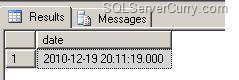### SQL Server: Convert to DateTime from other Datatypes

In this post, we will see how to convert data of different datatypes to a DateTime datatype, in SQL Server.

Sometimes date values that come from disparate sources may be of a different datatype other than DateTime such as int, varchar etc. In such cases we often need to convert the valuee back to DateTime. We will take two common scenarios of converting Int and Varchar datatype to DateTime

Consider the following examples:

Method 1 : Big Integer to Datetime

Assume that the date value along with time part is stored in Bigint datatype, use this query

Here’s the same query for you to try out:

declare @date bigint
set @date=20101219201119

select
cast(left(date,8)+' '+stuff(stuff(substring(date,9,6),5,0,':'),3,0,':') as datetime) from
(
select cast(@date as varchar(20)) as date
) as t

In the above example, the first 8 numbers denote a date and rest of numbers denote time values in the format HHMMSS. In the above code, left(date,8) extracts the date value. In order to have a proper date format we need to add ‘:’ in each of the time parts (hour, minute and second). The stuff function is used to add ‘:’ in the 3rd and 5th position and the entire string is converted to DateTime.

Method 2 : Varchar to Datetime

Assume that date value along with time part is stored in a Varchar datatype and a space seperates the date value from time values. Use this query:

Here’s the same query for you to try out:

declare @date varchar(20)
set @date='20101219 201119'

select
cast(left(@date,8)+ ' ' +
stuff(stuff(substring(@date,10,6),5,0,':'),3,0,':') as datetime)

OUTPUT